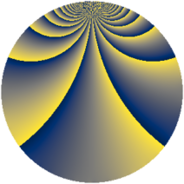# Properties

 Label 2001.2.oLevel $2001$ Weight $2$ Character orbit 2001.o Rep. character $\chi_{2001}(689,\cdot)$ Character field $\Q(\zeta_{14})$ Dimension $1416$ Sturm bound $480$

# Related objects

## Defining parameters

 Level: $$N$$ $$=$$ $$2001 = 3 \cdot 23 \cdot 29$$ Weight: $$k$$ $$=$$ $$2$$ Character orbit: $$[\chi]$$ $$=$$ 2001.o (of order $$14$$ and degree $$6$$) Character conductor: $$\operatorname{cond}(\chi)$$ $$=$$ $$2001$$ Character field: $$\Q(\zeta_{14})$$ Sturm bound: $$480$$

## Dimensions

The following table gives the dimensions of various subspaces of $$M_{2}(2001, [\chi])$$.

Total New Old
Modular forms 1464 1464 0
Cusp forms 1416 1416 0
Eisenstein series 48 48 0

## Trace form

 $$1416q - 14q^{3} - 252q^{4} - 28q^{6} - 2q^{9} + O(q^{10})$$ $$1416q - 14q^{3} - 252q^{4} - 28q^{6} - 2q^{9} - 40q^{13} - 260q^{16} - 14q^{18} - 74q^{24} - 248q^{25} + 28q^{27} - 28q^{31} - 40q^{36} - 56q^{39} + 63q^{48} + 112q^{49} + 16q^{52} + 42q^{54} - 84q^{55} - 90q^{58} - 110q^{64} + 49q^{69} - 56q^{72} - 28q^{73} - 48q^{78} + 30q^{81} + 32q^{82} + 252q^{85} - 14q^{87} - 62q^{93} + 64q^{94} - 51q^{96} + O(q^{100})$$

## Decomposition of $$S_{2}^{\mathrm{new}}(2001, [\chi])$$ into newform subspaces

The newforms in this space have not yet been added to the LMFDB.